# CBSE Important Questions

## Mathmatics Class IX

Q. 1. Visualize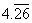on the number line up to four decimal places.

Q. 2. Show how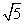can be represented on the number line .

Or

Represent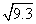on number line .

Q. 3. Represent the following numbers in the form of p/q :

1.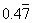2.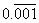Q. 4. (a). Find the value of following :

1. (32)2/5
2. (16)3/4

(b). Simplify the following :-

1.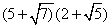Q. 5. Find five rational number between 1/2 & 1/3 ?

Q. 6. (a). Write three numbers whose decimal expansions are non terminating non recurring ?

(b). State whether True or false ? Give reasons for your answer :

1. Every whole number is a natural number.
2. Every integer is a rational number.
3. Every rational number is an integer.

Q. 7. Show that 1.272727=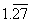can be expressed in the form of p/q, where p & q are integers & q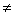0.

Q. 8. (a). Give an example of two irrational number whose produce is a rational number.

1. Comment on the nature of decimal expansion of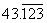& identify whether it is rational or irrational.

Q. 9. Find the value of ‘a’ & ‘b’ if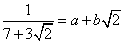Q. 10. Find three irrational number between the rational numbers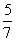&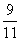?